## RS Aggarwal Class 6 Solutions Chapter 13 Angles and Their Measurement Ex 13B

These Solutions are part of RS Aggarwal Solutions Class 6. Here we have given RS Aggarwal Solutions Class 6 Chapter 13 Angles and Their Measurement Ex 13B.

Other Exercises

Question 1.
Solution:
(i) Obtuse angle
(ii) Right angle
(iii) straight angle
(iv) Reflex angle
(v) Acute angle
(vi) Complete angle

Question 2.
Solution:
We know that an acute angle is less than 90°
(ii) a right angle is equal to 90°
(iii) an obtuse angle is greater than 90° but less than 180°
(iv) an angle equal to 180° is a straight angle
(v) angle greater than 180° but less than 360° is called a reflex angle
(vi) angle equal to 360° is called a complete angle and angle equal to 0° is called a zero angle. Now the angles are :
(i) acute
(ii) obtuse
(iii) obtuse
(iv) right
(v) reflex
(vi) complete
(vii) obtuse
(viii) obtuse
(ix) acute
(x) acute
(xi) zero
(xii) acute Ans.

Question 3.
Solution:
(i) One right angle = 90°
(ii) Two right angles = (2 x 90)° = 180°
(iii) Three right angles = (3 x 90)° = 270°
(iv) Four right angles = (4 x 90)° = 360°
(v) $$\frac { 2 }{ 3 }$$ right angle = $$\left( \frac { 2 }{ 3 } \times { 90 }^{ O } \right)$$ = 60°
(vi) 1½ right angle = $$\left( 1\frac { 1 }{ 2 } \times { 90 }^{ O } \right)$$
$$\left( \frac { 3 }{ 2 } \times { 90 }^{ O } \right)$$ = 135°

Question 4.
Solution:
(i) When it is 3 o’ clock, the minute hand is at 12, and hour hand is at 3 as shown in the figure, clearly, the angle between the two hands 90°.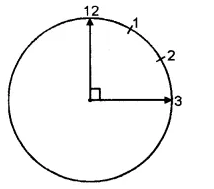(ii) When it is 6 o’ clock, the minute hand is at 12 and the hour hand is at 6 as shown in the figure. Clearly, the angle between the two hands of the clock is a straight angle is i.e. 180°.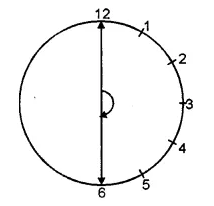(iii) When it is 12 o’ clock, both the hands of the clock lie at 12 as shown in the figure. Clearly, the angle between the two hands = 0°.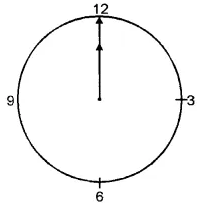(iv) When it is 9 o’ clock, the minute hand is at 12 and the hour hand is at 9 as shown in the figure. Clearly, the angle between the two hands = 90°.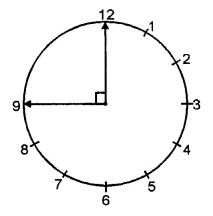Question 5.
Solution:
(i) Take the rular and draw any ray OA. Again using the rular, starting from O, draw a ray OB in such a way that the angle formed is less than 90°. Then, ∠AOB is the required acute angle.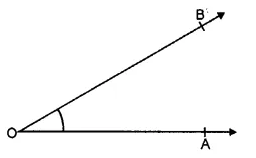(ii) Take the rular and draw any ray OA. Now, starting from O, draw another ray OB, with the help of the rular, such that the angle formed is greater than a right angle.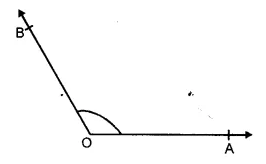Then, ∠AOB is the required obtuse angle.
(iii) Take a rular and draw any ray OA. Now, starting from O, draw ray OB in the opposite direction of the ray OA. Then ∠AOB is the required straight angle.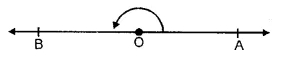Hope given RS Aggarwal Solutions Class 6 Chapter 13 Angles and Their Measurement Ex 13B are helpful to complete your math homework.

If you have any doubts, please comment below. Learn Insta try to provide online math tutoring for you.